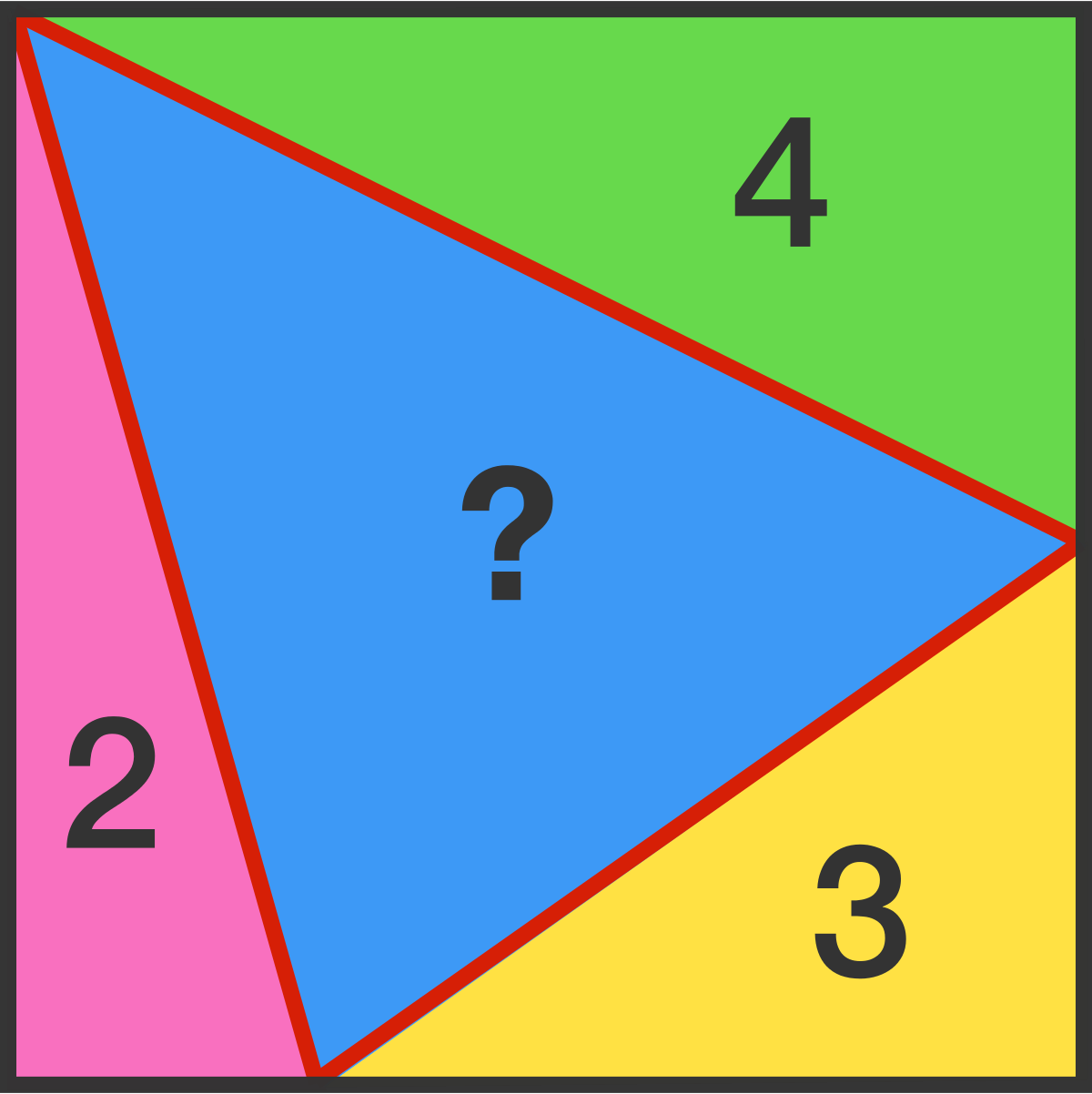Cody

# Problem 44267. Brain Teaser Solver

Write an algorithm to solve this brain teaser:

You have a square that is broken into four triangles, the area of three of those triangles is given. You must determine the area of the center triangle. Your function will take in 3 arguments A, B, and C. Below is an image of this problem, in this example A = 4, B = 3, and C = 2.### Solution Stats

36.84% Correct | 63.16% Incorrect
Last Solution submitted on Oct 31, 2019